1月8日，长城汽车2018年12月份销量数据重磅出炉。数据显示，12月，长城汽车销量高达133,794辆，销量再度突破100万辆。其中哈弗品牌12月销量再创新高，销量高达107,578辆，持续保持稳健增长。至此，哈弗品牌2018年累计销量高达766,062辆，连续四个月实现逆势增涨，强势助推哈弗品牌全球累计销量成功突破500万辆，成为首个以最快速度达到500万辆的中国SUV品牌。具体来说，“国民神车”哈弗H6的销量表现依然抢眼，12月销量达到50,303辆，累计67个月排名SUV销量第一，继续担当中国SUV的领军角色。同时哈弗H2、哈弗M6一如既往销量持续破万，分别销售11,772辆、15,042辆。此外，刚上市不久的哈弗F5和哈弗F7表现强劲，销量双双破万，合力为哈弗品牌贡献了21,175辆的销量，由此可见哈弗F系产品力及定位已初步赢得市场认可。随着哈弗旗下明星车型在销量上持续“多点开花”，哈弗品牌再次夺得中国SUV销量冠军，创下了连续9年蝉联中国SUV销量第一的记录。未来随着H、F双系的持续发力，哈弗品牌将再创辉煌。

逆势高涨，王者哈弗势不可挡，喜迎全球500万

在2018年车市微增长的态势下，哈弗品牌始终保持着稳健的增长步伐，连创佳绩。这不仅助力哈弗2018年交出了一份亮眼成绩单，也进一步彰显其强大的市场统治力优势，尽显哈弗品牌作为SUV领导者的王者风范。

哈弗取得如此耀眼的成绩绝非巧合。归根究底，得益于哈弗品牌自身的前瞻性定位、精准洞察以及不断优化的体系实力。自诞生之初，哈弗品牌就凭借着前瞻性的国际化视野，以及对市场和消费者精准洞察，提出了 “聚焦SUV”战略，致力于为消费者提供高品质SUV产品。多年来，在聚焦战略的指引下，哈弗品牌积极求变，不断自我突破，从产品，到服务，再到营销，建立起了一套极具竞争力的“体系实力”。这使得哈弗始终保持着强劲的增长势头，销量一路长虹，创下了连续9年稳居中国SUV销量榜首的车坛神话，成为引领自主品牌集体向上的领军者。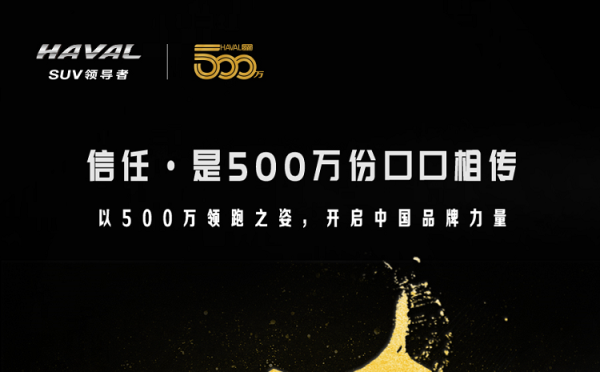如今，随着销量的节节攀升，哈弗品牌全球喜迎500万，不仅对于哈弗品牌来说是一次彰显霸主地位的重大历史时刻，同时作为中国首个突破500万的SUV单品牌，对于中国整个车市同样是值得纪念的大事件，象征着中国自主品牌的崛起势不可挡，为哈弗2018年画上了一个完美的句号。

荣誉与销量齐飞，哈弗2018年满载而归

2018年，在全球累计销量成功突破500万的同时，哈弗品牌的产品和品牌价值也得到了进一步提升，助力哈弗品牌在2018年斩获多项业内权威大奖。

品牌方面，哈弗自诞生以来始终坚持自主创新领先原则，不断提升品牌实力，这也为它赢得了国际化的至高荣誉。2018年，哈弗在国际权威品牌机构Brand Finance发布的“2018全球最有价值的100个汽车品牌”排行榜中位列第16名，超越法拉利、特斯拉等世界知名品牌，并一举成为最具价值中国汽车品牌第一名。这一傲人成绩的获得，不仅突显了哈弗是中国SUV市场名副其实的霸主，更证明了哈弗品牌的全球影响力在不断提升。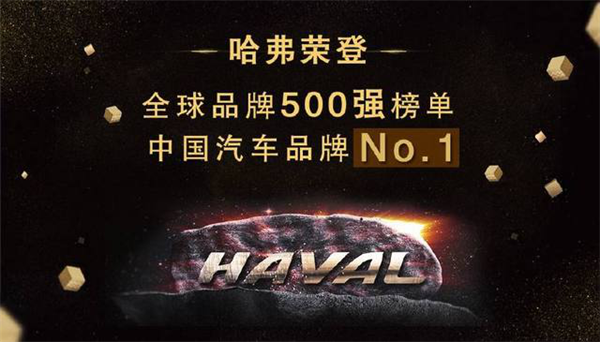产品方面，凭借着卓越的品质实力，哈弗SUV先后斩获多个国内外知名机构的大奖，涵盖了动力、操控、安全、满意度等多个维度，成为消费者的品质之选。更值得一提的是，哈弗在2018年还屡获国内外政府采购大单，进一步夯实了哈弗SUV的市场领先地位，全面彰显了哈弗SUV无可撼动的霸主地位。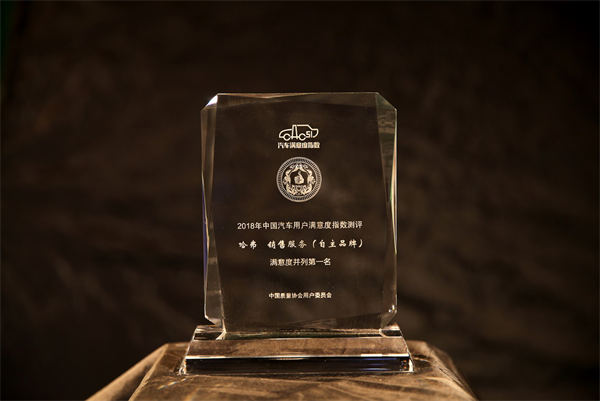随着哈弗品牌在2018年斩获多项权威大奖及销量一路飘红，哈弗2018年满载而归，不仅用实力证明了其SUV领导者的地位无可撼动，更是为哈弗实现全球500万销量奠定了坚实基础。

放眼全球，坚持创新引领，加速实现全球SUV第一梦

当下，随着中国SUV市场趋于饱和以及购车群体的日益年轻化，未来车市的新机遇在于对细分市场的深耕。因此，如何满足年轻人追求时尚与个性的需求，就成了未来车企在激烈竞争中取胜的关键因素。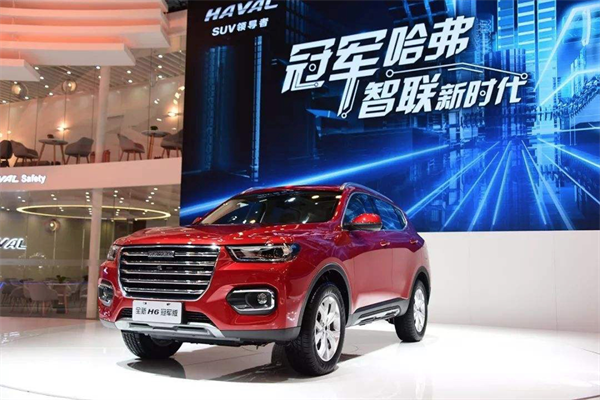面对愈发白热化的市场竞争，未来，哈弗将继续在“聚焦”战略的指引下，以SUV领导者格局放眼全球，以匠心精神为核心驱动力，凭借敏锐的市场嗅觉和强大的技术储备，主动出击，全力推进年轻化、智能化战略，大力发展智能技术，打造高品质、高性价比移动智能终端，以基础技术、用户思维、个性化服务，三位一体构建全场景化智能服务体系，为用户打造更智能、便利的产品体验，并逐步实现全线产品智能化，全面开启哈弗“智能新时代”。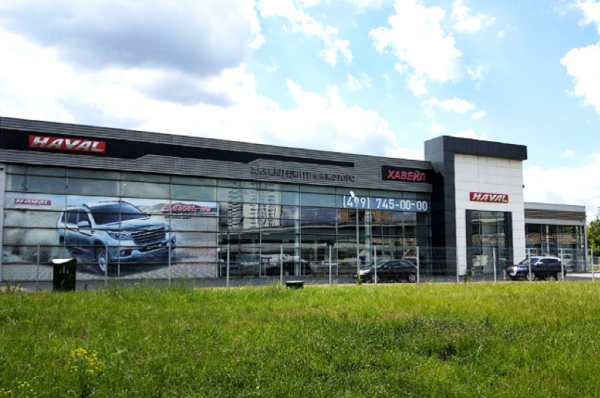同时，在产品布局上，通过H&F 双系战略，哈弗将进一步拓展细分市场，不断满足消费者愈发多元化的用车需求，致力成为全球领先的 SUV 品牌。此外，哈弗坚持国际化战略，构建以保定总部为核心，涵盖欧洲、亚洲、北美等全球研发布局。并不断扩大海外市场销售网络，以更强的市场渗透力强势打开国际市场，向世界展示自主品牌势不可挡的崛起之势。

结语：

2018年，市场在变化，需求在升级，机遇与挑战并存。作为SUV领导者，哈弗凭借着对市场与消费者的深入精准洞察，在“聚焦SUV”战略的指引下，顺势而为，逆势向上，不断突破自我，全球累计销量突破500万辆，成为首个以最快速度达到500万辆的中国SUV品牌，充分彰显全球消费者对于哈弗品牌的高度认可，也象征着中国自主品牌的崛起势不可挡。这一重大历史时刻，对于哈弗品牌来说，是一个阶段性的胜利，更开启了哈弗品牌的新篇章。

未来，随着哈弗在年轻化、智能化以及全球化布局等方面的全力出击，哈弗品牌在实现全球SUV领导者的道路上将越走越坚定，一路勇往直前。

2018，巅峰之路，“王者”哈弗从未止步。2019，哈弗将再创辉煌！

##### 相关资讯

|分享

•已阅！握爪
•我手滑为你点赞
•128个赞！
•不明觉厉
•阅后既醉
•有钱！任性
•照片太美，我不敢看
•干货！杠杠的
•高大上
•膜拜中！
•请接受我的膝盖
•猫哥/猫妹么么哒
•神吐槽
•我只笑笑不说话
•我想静静
•窒息
##### 资讯相关车系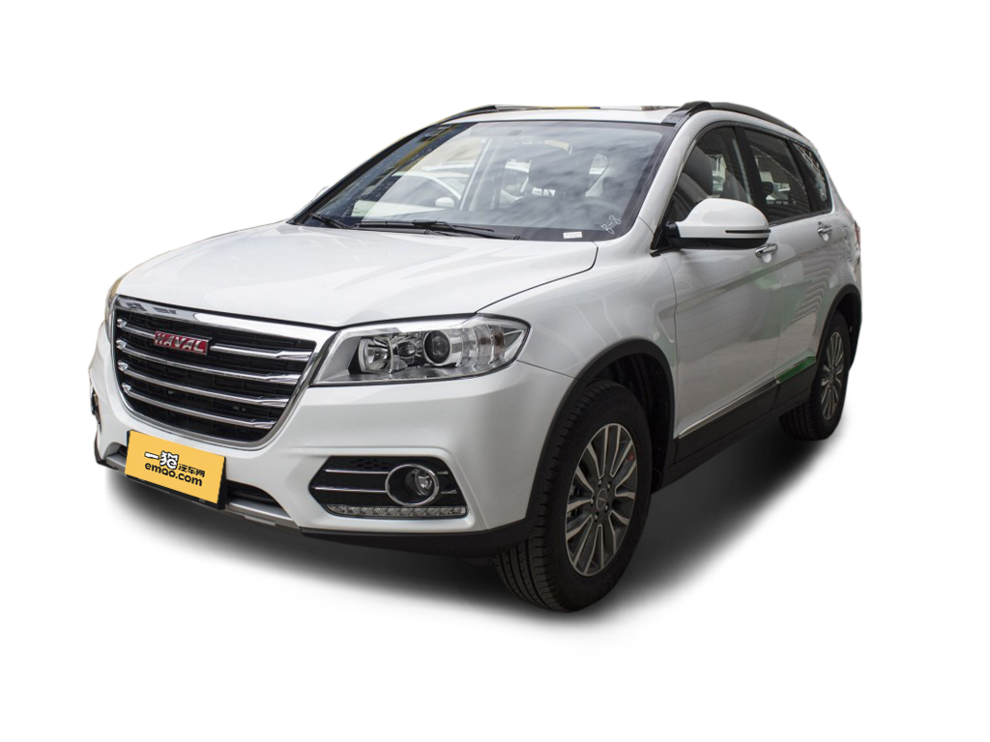##### 热度排行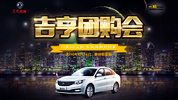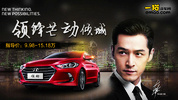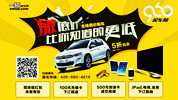• 阿斯顿·马丁
• 奥迪
• 阿尔法罗密欧
• ALPINA
• 爱驰
• AC Schnitzer

• 巴博斯
• 宝骏
• 宝马
• 保时捷
• 北汽制造
• 奔驰
• 奔腾
• 本田
• 比亚迪
• 标致
• 别克
• 宾利
• 布加迪
• 北汽威旺
• 北京
• 北汽绅宝
• 北汽幻速
• 北汽新能源
• 宝沃
• 比速汽车
• 北汽道达
• 北京汽车
• 比德文汽车
• 铂驰
• 北汽昌河

• 昌河
• 长安
• 长城
• 长安商用
• 成功汽车
• 长江EV
• 长安轻车型
• 长安欧尚
• 长安凯程
• 长安跨越
• 车驰汽车

• 大众
• 道奇
• 东风
• 东风风神
• 东风小康
• 东南
• DS
• 东风风行
• 东风风度
• 东风风光
• 电咖
• 大乘汽车
• 大运
• 东风富康

• 法拉利
• 菲亚特
• 丰田
• 福迪
• 福特
• 福田汽车
• 福汽启腾
• 风诺
• 福田

• GMC
• 广汽传祺
• 广汽吉奥
• 观致
• 国金汽车
• 广汽新能源
• 广汽集团
• 国机智骏

• 哈飞
• 海格
• 海马
• 华泰
• 黄海
• 恒天
• 红旗
• 哈弗
• 华颂
• 华凯
• 华泰新能源
• 汉腾汽车
• 华利
• 红星汽车
• HYCAN合创
• 汉龙汽车

• Jeep
• 江淮
• 江铃
• 捷豹
• 金杯
• 九龙
• 吉利汽车
• 金旅
• 金龙
• 江铃集团轻汽
• 江铃集团新能源
• 君马汽车
• 捷途
• 钧天
• 捷达
• 几何汽车

• 开瑞
• 凯迪拉克
• 科尼赛克
• 克莱斯勒
• KTM
• 卡威
• 凯翼
• 康迪
• 康迪电动汽车集团
• 开沃汽车
• 卡升

• 猎豹汽车
• 兰博基尼
• 劳斯莱斯
• 雷克萨斯
• 雷诺
• 理念
• 力帆
• 莲花汽车
• 林肯
• 铃木
• 陆风
• 路虎
• 路特斯
• 领志
• 领克
• 零跑汽车
• Lorinser
• LOCAL MOTORS
• LITE
• 领途汽车
• 理想汽车
• 罗夫哈特
• 力帆汽车

• MG
• MINI
• 马自达
• 玛莎拉蒂
• 迈凯伦
• 摩根
• 名爵
• 迈莎锐
• 迈迈

• 纳智捷
• 哪吒汽车

• 讴歌
• 欧宝
• 欧朗
• 欧拉
• 欧尚汽车

• 帕加尼
• Polestar极星

• 奇瑞
• 启辰
• 起亚
• 前途
• 庆铃汽车
• 骐铃汽车
• 全球鹰
• 乔治·巴顿

• 日产
• 荣威
• 瑞麒汽车
• 如虎
• 瑞驰
• 瑞麒
• 瑞驰新能源
• 容大智造

• 上汽大通
• smart
• 三菱
• 双环
• 双龙
• 斯巴鲁
• 斯柯达
• 萨博
• 思铭
• 赛麟
• SWM斯威汽车
• 上汽MAXUS
• SRM鑫源
• 思皓
• SHELBY
• 上喆

• TESLA
• 泰卡特
• 腾势
• 特斯拉
• 天际汽车

• 威麟
• 威兹曼
• 沃尔沃
• 五菱汽车
• 五十铃
• 潍柴英致
• WEY
• 蔚来
• 威马汽车
• 潍柴汽车

• 现代
• 雪佛兰
• 雪铁龙
• 西雅特
• 新特汽车
• 小鹏汽车
• 新宝骏

• 野马汽车
• 一汽
• 依维柯
• 英菲尼迪
• 永源
• 驭胜
• 云度
• 宇通客车
• 云雀汽车
• 远程汽车
• 一汽凌河

• 中华
• 中兴
• 众泰
• 知豆
• 之诺
• 正道汽车
• A
• B
• C
• D
• E
• F
• G
• H
• I
• J
• K
• L
• M
• N
• O
• P
• Q
• R
• S
• T
• U
• V
• W
• X
• Y
• Z

• 阿斯顿·马丁
• 奥迪
• 阿尔法罗密欧
• ALPINA
• 爱驰
• AC Schnitzer

• 巴博斯
• 宝骏
• 宝马
• 保时捷
• 北汽制造
• 奔驰
• 奔腾
• 本田
• 比亚迪
• 标致
• 别克
• 宾利
• 布加迪
• 北汽威旺
• 北京
• 北汽绅宝
• 北汽幻速
• 北汽新能源
• 宝沃
• 比速汽车
• 北汽道达
• 北京汽车
• 比德文汽车
• 铂驰
• 北汽昌河

• 昌河
• 长安
• 长城
• 长安商用
• 成功汽车
• 长江EV
• 长安轻车型
• 长安欧尚
• 长安凯程
• 长安跨越
• 车驰汽车

• 大众
• 道奇
• 东风
• 东风风神
• 东风小康
• 东南
• DS
• 东风风行
• 东风风度
• 东风风光
• 电咖
• 大乘汽车
• 大运
• 东风富康

• 法拉利
• 菲亚特
• 丰田
• 福迪
• 福特
• 福田汽车
• 福汽启腾
• 风诺
• 福田

• GMC
• 广汽传祺
• 广汽吉奥
• 观致
• 国金汽车
• 广汽新能源
• 广汽集团
• 国机智骏

• 哈飞
• 海格
• 海马
• 华泰
• 黄海
• 恒天
• 红旗
• 哈弗
• 华颂
• 华凯
• 华泰新能源
• 汉腾汽车
• 华利
• 红星汽车
• HYCAN合创
• 汉龙汽车

• Jeep
• 江淮
• 江铃
• 捷豹
• 金杯
• 九龙
• 吉利汽车
• 金旅
• 金龙
• 江铃集团轻汽
• 江铃集团新能源
• 君马汽车
• 捷途
• 钧天
• 捷达
• 几何汽车

• 开瑞
• 凯迪拉克
• 科尼赛克
• 克莱斯勒
• KTM
• 卡威
• 凯翼
• 康迪
• 康迪电动汽车集团
• 开沃汽车
• 卡升

• 猎豹汽车
• 兰博基尼
• 劳斯莱斯
• 雷克萨斯
• 雷诺
• 理念
• 力帆
• 莲花汽车
• 林肯
• 铃木
• 陆风
• 路虎
• 路特斯
• 领志
• 领克
• 零跑汽车
• Lorinser
• LOCAL MOTORS
• LITE
• 领途汽车
• 理想汽车
• 罗夫哈特
• 力帆汽车

• MG
• MINI
• 马自达
• 玛莎拉蒂
• 迈凯伦
• 摩根
• 名爵
• 迈莎锐
• 迈迈

• 纳智捷
• 哪吒汽车

• 讴歌
• 欧宝
• 欧朗
• 欧拉
• 欧尚汽车

• 帕加尼
• Polestar极星

• 奇瑞
• 启辰
• 起亚
• 前途
• 庆铃汽车
• 骐铃汽车
• 全球鹰
• 乔治·巴顿

• 日产
• 荣威
• 瑞麒汽车
• 如虎
• 瑞驰
• 瑞麒
• 瑞驰新能源
• 容大智造

• 上汽大通
• smart
• 三菱
• 双环
• 双龙
• 斯巴鲁
• 斯柯达
• 萨博
• 思铭
• 赛麟
• SWM斯威汽车
• 上汽MAXUS
• SRM鑫源
• 思皓
• SHELBY
• 上喆

• TESLA
• 泰卡特
• 腾势
• 特斯拉
• 天际汽车

• 威麟
• 威兹曼
• 沃尔沃
• 五菱汽车
• 五十铃
• 潍柴英致
• WEY
• 蔚来
• 威马汽车
• 潍柴汽车

• 现代
• 雪佛兰
• 雪铁龙
• 西雅特
• 新特汽车
• 小鹏汽车
• 新宝骏

• 野马汽车
• 一汽
• 依维柯
• 英菲尼迪
• 永源
• 驭胜
• 云度
• 宇通客车
• 云雀汽车
• 远程汽车
• 一汽凌河

• 中华
• 中兴
• 众泰
• 知豆
• 之诺
• 正道汽车
• A
• B
• C
• D
• E
• F
• G
• H
• I
• J
• K
• L
• M
• N
• O
• P
• Q
• R
• S
• T
• U
• V
• W
• X
• Y
• Z

• 阿斯顿·马丁
• 奥迪
• 阿尔法罗密欧
• ALPINA
• 爱驰
• AC Schnitzer

• 巴博斯
• 宝骏
• 宝马
• 保时捷
• 北汽制造
• 奔驰
• 奔腾
• 本田
• 比亚迪
• 标致
• 别克
• 宾利
• 布加迪
• 北汽威旺
• 北京
• 北汽绅宝
• 北汽幻速
• 北汽新能源
• 宝沃
• 比速汽车
• 北汽道达
• 北京汽车
• 比德文汽车
• 铂驰
• 北汽昌河

• 昌河
• 长安
• 长城
• 长安商用
• 成功汽车
• 长江EV
• 长安轻车型
• 长安欧尚
• 长安凯程
• 长安跨越
• 车驰汽车

• 大众
• 道奇
• 东风
• 东风风神
• 东风小康
• 东南
• DS
• 东风风行
• 东风风度
• 东风风光
• 电咖
• 大乘汽车
• 大运
• 东风富康

• 法拉利
• 菲亚特
• 丰田
• 福迪
• 福特
• 福田汽车
• 福汽启腾
• 风诺
• 福田

• GMC
• 广汽传祺
• 广汽吉奥
• 观致
• 国金汽车
• 广汽新能源
• 广汽集团
• 国机智骏

• 哈飞
• 海格
• 海马
• 华泰
• 黄海
• 恒天
• 红旗
• 哈弗
• 华颂
• 华凯
• 华泰新能源
• 汉腾汽车
• 华利
• 红星汽车
• HYCAN合创
• 汉龙汽车

• Jeep
• 江淮
• 江铃
• 捷豹
• 金杯
• 九龙
• 吉利汽车
• 金旅
• 金龙
• 江铃集团轻汽
• 江铃集团新能源
• 君马汽车
• 捷途
• 钧天
• 捷达
• 几何汽车

• 开瑞
• 凯迪拉克
• 科尼赛克
• 克莱斯勒
• KTM
• 卡威
• 凯翼
• 康迪
• 康迪电动汽车集团
• 开沃汽车
• 卡升

• 猎豹汽车
• 兰博基尼
• 劳斯莱斯
• 雷克萨斯
• 雷诺
• 理念
• 力帆
• 莲花汽车
• 林肯
• 铃木
• 陆风
• 路虎
• 路特斯
• 领志
• 领克
• 零跑汽车
• Lorinser
• LOCAL MOTORS
• LITE
• 领途汽车
• 理想汽车
• 罗夫哈特
• 力帆汽车

• MG
• MINI
• 马自达
• 玛莎拉蒂
• 迈凯伦
• 摩根
• 名爵
• 迈莎锐
• 迈迈

• 纳智捷
• 哪吒汽车

• 讴歌
• 欧宝
• 欧朗
• 欧拉
• 欧尚汽车

• 帕加尼
• Polestar极星

• 奇瑞
• 启辰
• 起亚
• 前途
• 庆铃汽车
• 骐铃汽车
• 全球鹰
• 乔治·巴顿

• 日产
• 荣威
• 瑞麒汽车
• 如虎
• 瑞驰
• 瑞麒
• 瑞驰新能源
• 容大智造

• 上汽大通
• smart
• 三菱
• 双环
• 双龙
• 斯巴鲁
• 斯柯达
• 萨博
• 思铭
• 赛麟
• SWM斯威汽车
• 上汽MAXUS
• SRM鑫源
• 思皓
• SHELBY
• 上喆

• TESLA
• 泰卡特
• 腾势
• 特斯拉
• 天际汽车

• 威麟
• 威兹曼
• 沃尔沃
• 五菱汽车
• 五十铃
• 潍柴英致
• WEY
• 蔚来
• 威马汽车
• 潍柴汽车

• 现代
• 雪佛兰
• 雪铁龙
• 西雅特
• 新特汽车
• 小鹏汽车
• 新宝骏

• 野马汽车
• 一汽
• 依维柯
• 英菲尼迪
• 永源
• 驭胜
• 云度
• 宇通客车
• 云雀汽车
• 远程汽车
• 一汽凌河

• 中华
• 中兴
• 众泰
• 知豆
• 之诺
• 正道汽车
• A
• B
• C
• D
• E
• F
• G
• H
• I
• J
• K
• L
• M
• N
• O
• P
• Q
• R
• S
• T
• U
• V
• W
• X
• Y
• Z